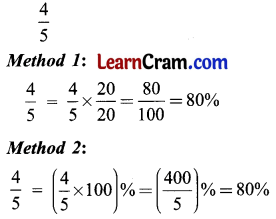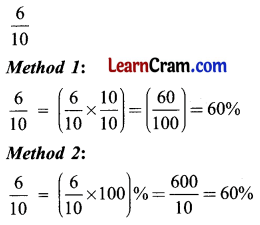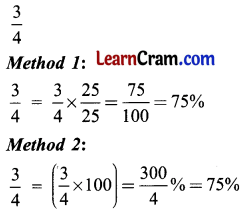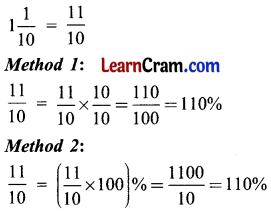# DAV Class 5 Maths Chapter 12 Worksheet 3 Solutions

The DAV Books Solutions Class 5 Maths and DAV Class 5 Maths Chapter 12 Worksheet 3 Solutions of Percentage offer comprehensive answers to textbook questions.

## DAV Class 5 Maths Ch 12 Worksheet 3 Solutions

Question 1.
Convert the following fractions into percentages.
(a) $$\frac{4}{5}$$
Solution:(b) $$\frac{6}{10}$$
Solution:(c) $$\frac{3}{4}$$
Solution:(d) $$\frac{9}{50}$$
Solution:(e) 1$$\frac{2}{5}$$
Solution:(f) 1$$\frac{1}{10}$$
Solution:Question 2.
Convert the following into percentages.
(a) 6
Solution:
Method 1:
6 = 6 × $$\frac{100}{100}$$
= $$\frac{600}{100}$$
= 600%
Method 2:
6 = (6 × 100)% = 600%

(b) 15
Solution:
Method 1:
15 = 15 × $$\frac{100}{100}$$
= $$\frac{1500}{100}$$
= 1500%
Method 2:
15 = (15 × 100)% = 1500%(c) 28
Solution:
Method 1:
28 = 28 × $$\frac{100}{100}$$
= $$\frac{2800}{100}$$
= 2800%
Method 2:
28 = (28 × 100)% = 2800%

(d) 13
Solution:
Method 1:
13 = 13 × $$\frac{100}{100}$$
= $$\frac{1300}{100}$$
= 1300%
Method 2:
13 = (13 × 100)% = 1300%

(e) 9
Solution:
Method 1:
9 = 9 × $$\frac{100}{100}$$
= $$\frac{900}{100}$$
= 900%
Method 2:
9 = (9 × 100)% = 900%

(f) 1
Solution:
Method 1:
1 = 1 × $$\frac{100}{100}$$
= $$\frac{100}{100}$$
= 100%
Method 2:
1 = (1 × 100)% = 100%Question 3.
Convert the following decimals into percentages.
(a) 0.45
Solution:
Method 1:
0. 45 = $$\frac{45}{100}$$ = 45%
Method 2:
0. 45 = (0.45 × 100)% = 45%

(b) 0.09
Solution:
Method 1:
0.09 = $$\frac{9}{100}$$ = 9%
Method 2:
0.09 = (0.09 × 100)% = 9%

(c) 2.25
Solution:
Method 1:
2.25 = $$\frac{225}{100}$$ = 225%
Method 2:
2.25 = (2.25 × 100)% = 225%

(d) 7.6
Solution:
Method 1:
7.6 = $$\frac{76}{10} \times \frac{10}{10}$$
= $$\frac{760}{100}$$
= 760%
Method 2:
7.6 = (7.6 × 100)% = 760%

(e) 0.01
Solution:
Method 1:
0.01 = $$\frac{1}{100}$$ = 1%
Method 2:
0.01 = (0.01 × 100)% = 1%(f) 0.135
Solution:
Method 1:
0.135 = $$\frac{135}{1000}$$
= $$\frac{135 \div 10}{1000 \div 10}$$
= 13.5%
Method 2:
0.135 = (0.135 × 100)% = 13.5%

Question 4.
Choose the correct number.
(a) 5% = 5, 0.5, 0.05, 0.005
Solution:
0.05

(b) 45% = 4.5, 0.45, 0.045, 0.0045
Solution:
0.45

(c) 125% = 0.125, 12.5, 1.25, 125
Solution:
1.25

Question 5.
Solve the following questions.
(a) Reema scored 40 marks out of 50 in her Mathematics test. Find the percentage of marks scored by her.
Solution:
Reema scored = 40 marks
Maximum marks = 50 marks
Percentage of marks = ($$\frac{40}{50}$$ × 100)%
= (4 × 20)%
= 80%

(b) In a packet of 25 toffees, three toffees are mango bites. What is the percentage of mango bite toffees?
Solution:
Total toffees = 25
No. of mango bites = 3
% of mango bite toffees = ($$\frac{3}{25}$$ × 100)% = 12%(c) In the month of April, a school worked for only 24 days. What is the percentage of working days?
Solution:
Total no. of days in April = 30 days
Number of working days = 24 days
Percentage of working days = ($$\frac{24}{30}$$ × 100)% = 80%

(d) In an orchard, there are 250 trees. 175 of them are apple trees. Find the percentage of apple trees.
Solution:
Total number of trees = 250
No. of apple trees = 175
Percentage of apple trees = ($$\frac{175}{250}$$ × 100)% = 70%

DAV Class 5 Maths Chapter 12 Worksheet 3 Notes

Convert a fraction into a percentage.

Example 1.
Let us convert $$\frac{2}{5}$$ into percentage.
Solution:
Method 1:
$$\frac{2}{5}=\frac{2}{5} \times \frac{20}{20}=\frac{40}{100}$$ = 40%
Method 2:
$$\frac{2}{5}$$ = ($$\frac{2}{5}$$ × 100)%
= $$\frac{200}{5}$$%
= 40%

We can convert a fraction into a percentage by two methods:

• Convert a fraction into an equivalent fraction with denominator 100.
• Multiply the fraction by 100.Convert a decimal number into a percentage.

Example 2.
Convert 0.2 into a percentage.
Solution:
Method 1:
0.2 = $$\frac{2}{10}$$
= $$\frac{2 \times 10}{10 \times 10}$$
= $$\frac{20}{100}$$
= 20%
Method 2:
0.2 = (0.2 × 100)% = 20%

We can convert decimals into percentages by two methods:

• Convert decimal into an equivalent fraction with denominator 100.
• Multiply the decimal by 100.

Convert a whole number into a percentage.

Example 3.
Convert 3 into a percentage.
Solution:
Method 1:
3 = $$\frac{3 \times 100}{100}$$
= $$\frac{300}{100}$$
= 300%
Method 2:
3 = (3 × 100)% = 300%We can convert whole numbers into percentages by 2 methods:

• Convert whole numbers into equivalent fractions with denominator 100.
• Multiply the whole number by 100.